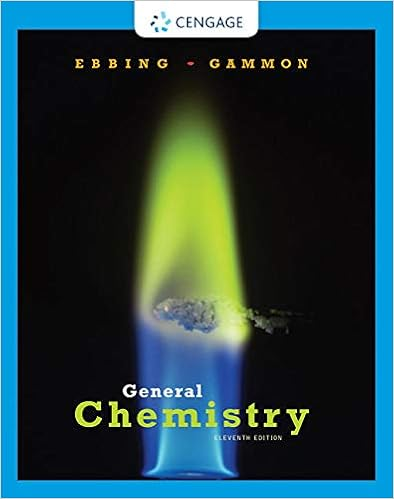# B 79500 250 318 c70 10 3 80 10 4 0007 00008 00062 62

• Homework Help
• 5
• 100% (3) 3 out of 3 people found this document helpful

This preview shows page 1 - 4 out of 5 pages.

##### We have textbook solutions for you!
The document you are viewing contains questions related to this textbook.The document you are viewing contains questions related to this textbook.
Chapter 1 / Exercise 1.100
General Chemistry
Ebbing/GammonExpert Verified
b.79,500 ÷ (2.5 × 102) = 79,500 ÷ 250 = 318 c.(7.0 × 10–3) – (8.0 × 10–4) = 0.007 – 0.0008 = 0.0062 = 6.2 x 10-3d.(1.0 × 104) × (9.9 × 106) = 1000 x 9900000 = 9.9 x 1010(Reference: Chang 1.31)4.What is the number of significant figures in each of the following measurements? (8 points)1Copyright © 2016 by Thomas Edison State University. All rights reserved.
##### We have textbook solutions for you!
The document you are viewing contains questions related to this textbook.The document you are viewing contains questions related to this textbook.
Chapter 1 / Exercise 1.100
General Chemistry
Ebbing/GammonExpert Verified
4
2
5
4
3
1
1
2
5.Express the results in the proper number of significant figures. (12 points)
6.Carry out the following conversions: (16 points)a.22.6 m to dm
b.25.4 mg to kilograms
c.556 mL to liters
d.10.6 kg/m3to g/cm3
•••